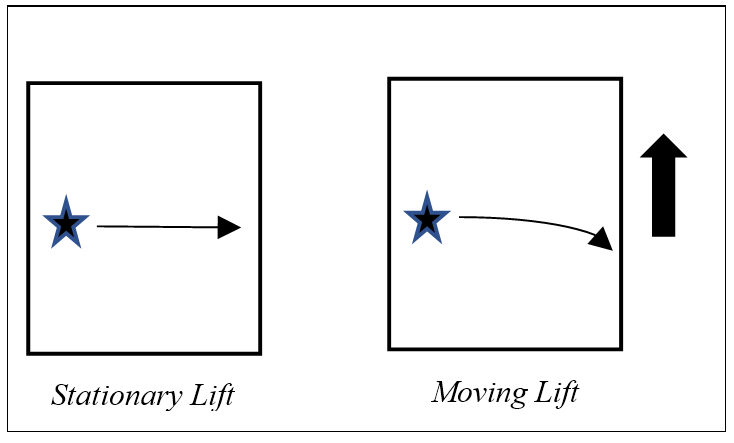# QR5.4.4 Gravity Bends LightFigure 5.8. Light bends when lift moves up

Einstein deduced that gravity bends light by imagining a flashlight shining horizontally in a lift accelerating upwards (Figure 5.8). As the lift accelerates up, the light curves relative to it, so if gravity is equivalent to acceleration then it should bend light. Light should “fall” by gravity just as matter does, and light passing the sun is indeed bent. The analogy worked but how does inert matter reach out to move massless light?

In quantum realism, the sun bends light passing it by the same quantum field that keeps the planets in a gravitational orbit. In these terms, the sun is so massive that its existence permeates the entire solar system as quantum processing spreading. Since a photon is also processing that spreads in every direction, as it passes the sun, the greater load nearer to the sun slows down the spread in that direction. The photon wave front is thus bent by gravity just as in refraction (3.6.2) light is bent by water that also slows it down. If one side of a light wave has a higher load, those slower transfers skew the light that way. Light has no mass but it has quantum processing, so the gravity gradient affects it.

Next